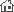Home | Products |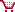How to buy

# Examples of A-PDF Watermark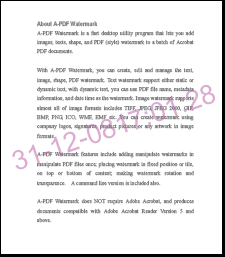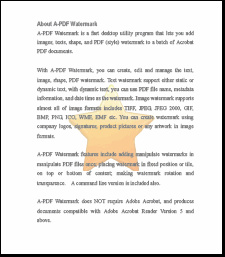Add background image into PDF Stretch Mode, Opacity = 80, Rotate = 0 Download the example PDF Add dynamic stamp(Date time) into PDF Fix Mode, Opacity = 80, Rotate = 30 Download the example PDF Add your logo image over pdf Fix Mode, Opacity = 80, Rotate = 0 Download the example PDF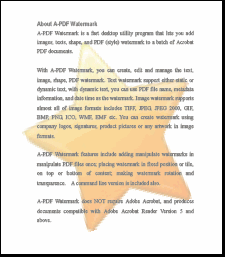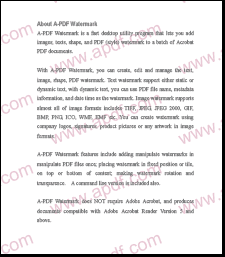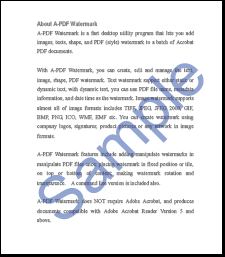Add your logo image over pdf Stretch Mode, Opacity = 80, Rotate = 15 Download the example PDF Watermark pdf using copyright information Tile Mode, Opacity = 80, Rotate = 45 Download the example PDF Put your sign on pdf Fix Mode, Opacity = 100, Rotate =45. Download the example PDF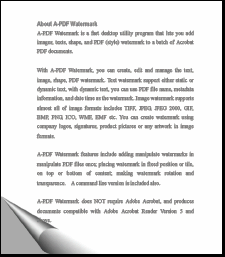Put your image(art-border) on pdf Stretch Mode, Opacity = 100, Rotate =0 Download the example PDF Put your image(art-border) on pdf str Mode, Opacity = 100, Rotate =0 Download the example PDF Put your image(art-border) on pdf Fix Mode, Opacity = 90, Rotate =0 Download the example PDF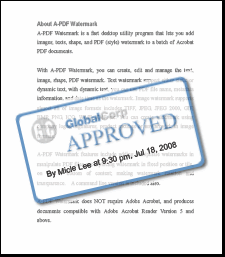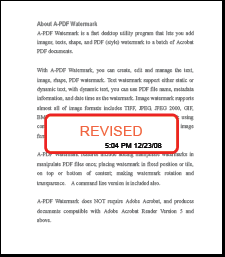Put your custom stamp on pdf Fix Mode, Opacity = 80, Rotate =15 Download the example PDF Put multiple stamp(text and image) over pdf Fix Mode: left-top, Opacity = 80, Rotate =0 Download the example PDF Put custom multiple stamp on pdf Fix Mode, Opacity = 0, Rotate =0 Download the example PDF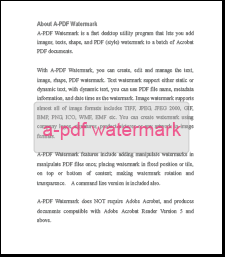Put Custom stamp(pdf content) over pdf Fix Mode, Opacity = 80, Rotate =45 Download the example PDF Put multiple stamp(text and shape) over pdf Fix Mode, left-top, Opacity = 80, Rotate =0 Download the example PDF Put Custom stamp(pdf content) over pdf Fix Mode, Opacity = 80, Rotate =45 Download the example PDF